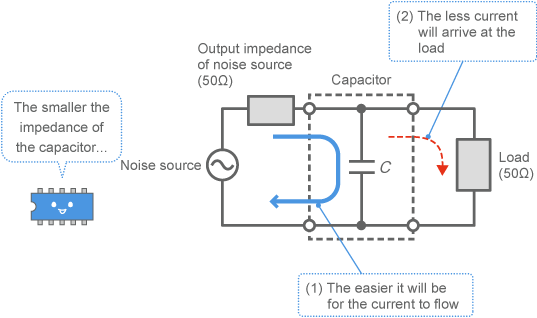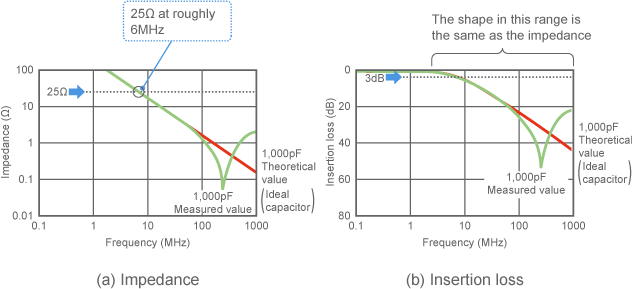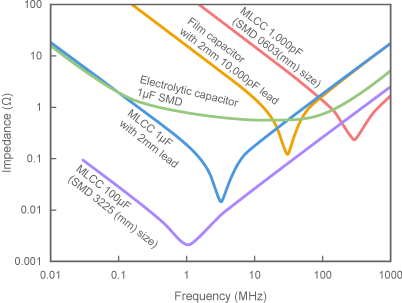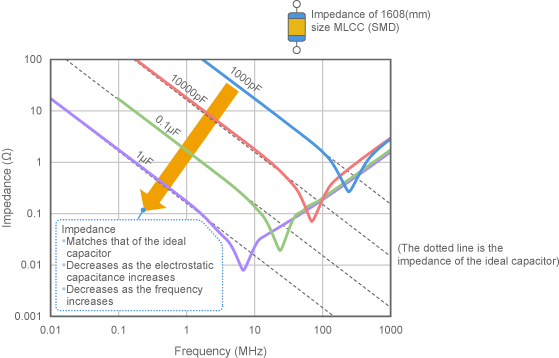Noise Suppression Products/EMI Suppression Filters

Noise Suppression Basic Course Section 1
Chapter 1
Chapter 2
Chapter 3
Chapter 4
Chapter 5
Chapter 6
Chapter 6

EMI suppression filters

6-5. Actual characteristics of capacitors

This section discusses why the noise reduction properties of a simple bypass capacitor differ from the basic characteristics. Knowing why this is can help you to construct filters that provide excellent noise reduction at lower costs, and to select parts with good cost-effectiveness.

6-5-1. Review of bypass capacitor operation

(1) Bypass the noise current to ground

Some noise reduction filters built from capacitors use bypass capacitors. As shown in Fig.
1, bypass capacitors eliminate noise by bypassing the noise current to the ground.Fig. 1 Bypass capacitor operation

(2) The smaller the impedance, the greater the noise reduction

The smaller the impedance of the bypass capacitor, the easier it will be for the current to flow ( (1) in Fig. 1) . This means that more noise will be eliminated. In other words, insertion loss is increased.
For example, if the insertion loss and impedance of the 1,000pF capacitor introduced in Section 6-4 are compared, the shape of the graphs will be just about the same, as shown in Fig.2. This is because an insertion loss of 3dB occurs at frequencies at which the impedance is 25 ohms or lower, while the insertion loss will be greater the lower the impedance is in frequencies above that range.Fig. 2 Relationship between capacitor impedance and insertion loss

(3) The noise reduction effect of a capacitor is expressed by its impedance

The noise reduction effect of a capacitor can therefore be expressed with impedance, in the attenuation range. To keep the explanation simple, this discussion will only take impedance into consideration.
You may be aware that the impedance of a capacitor is inversely proportional to the frequency and electrostatic capacitance. This is why, when graphed, impedance forms a simple downward sloping line, as shown by the theoretical values in Fig. 2 (a) . These theoretical values will be referred to as the "ideal capacitor" in future graphs, and will be used for comparison purposes.

(4) Examples of actual capacitor impedance measurements

Fig. 3 shows examples of the actual impedance measured from several capacitors. A film capacitor, some MLCCs, and an electrolytic capacitor are shown in the graph.
The MLCCs and film capacitor look similar as they all form rough V-shaped curves. The electrolytic capacitor forms a round U-shaped curve at the bottom. This shows that the trend shown by the 1,000pF capacitor in Fig. 2 is common to all capacitors. The reason for this shape is explained below.
Note however that the measured values used here are just some examples to demonstrate trends, and that values may differ depending on the product.Fig. 3 Examples of capacitor frequency characteristics

(5) The greater the electrostatic capacitance, the smaller the impedance

The following describes a case where the electrostatic capacitance is changed for a given type of capacitor.
Fig. 4 shows what happens when the electrostatic capacitance of an MLCC (1608 size SMD) is changed from 1,000pF to 1μF by a factor of ten each step (nominal values). The impedance of an ideal capacitor is shown with a dotted line for comparison.
As shown in the figure, the impedance of the capacitor forms a V-shaped curve that is very close to that of the ideal capacitor on the left side, and the lines for each electrostatic capacitance lined up cleanly in order. The capacitor can be seen as a simple electrostatic capacitance element at these frequencies.Fig. 4 Examples of MLCC impedance at each capacity (1608 size)

Top of page